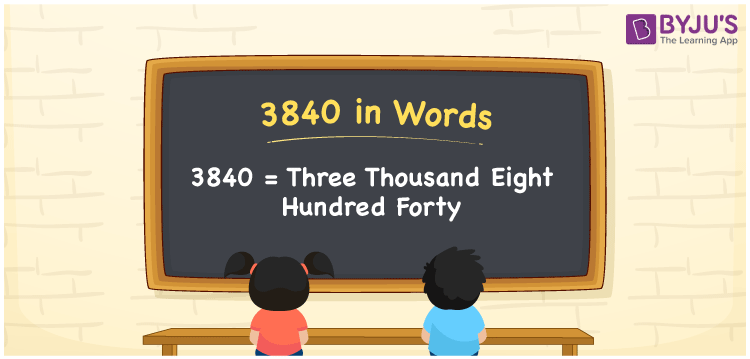# 3840 in Words

3840 in words can be written as Three Thousand Eight Hundred Forty. If you buy toys for Rs. 3840 for your kid, then you can say that “I have bought toys for Three Thousand Eight Hundred Forty Rupees for my kid”. Using the place value chart of 3840, convert the numbers into words effortlessly. Therefore, 3840 can be read as “Three Thousand Eight Hundred Forty” in words.

 3840 in words Three Thousand Eight Hundred Forty Three Thousand Eight Hundred Forty in Numbers 3840

## 3840 in English Words## How to Write 3840 in Words?

Four digits are present in 3840. Students can learn the place value of each digit using the table provided here.

 Thousands Hundreds Tens Ones 3 8 4 0

3840 in expanded form is:

3 x Thousand + 8 × Hundred + 4 × Ten + 0 × One

= 3 x 1000 + 8 × 100 + 4 × 10 + 0 × 1

= 3000 + 800 + 40

= 3840

= Three Thousand Eight Hundred Forty

Therefore, 3840 in words is written as Three Thousand Eight Hundred Forty.

3840 is a natural number that precedes 3841 and succeeds 3839.

3840 in words – Three Thousand Eight Hundred Forty

Is 3840 an odd number? – No

Is 3840 an even number? – Yes

Is 3840 a perfect square number? – No

Is 3840 a perfect cube number? – No

Is 3840 a prime number? – No

Is 3840 a composite number? – Yes

## Frequently Asked Questions on 3840 in Words

Q1

### Write 3840 in words.

3840 can be written in words as “Three Thousand Eight Hundred Forty”.
Q2

### How do you write Three Thousand Eight Hundred Forty in numbers?

Three Thousand Eight Hundred Forty can be written in numbers as 3840.
Q3

### Is 3840 an even number?

3840 is an even number as it is completely divisible by 2.